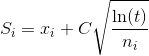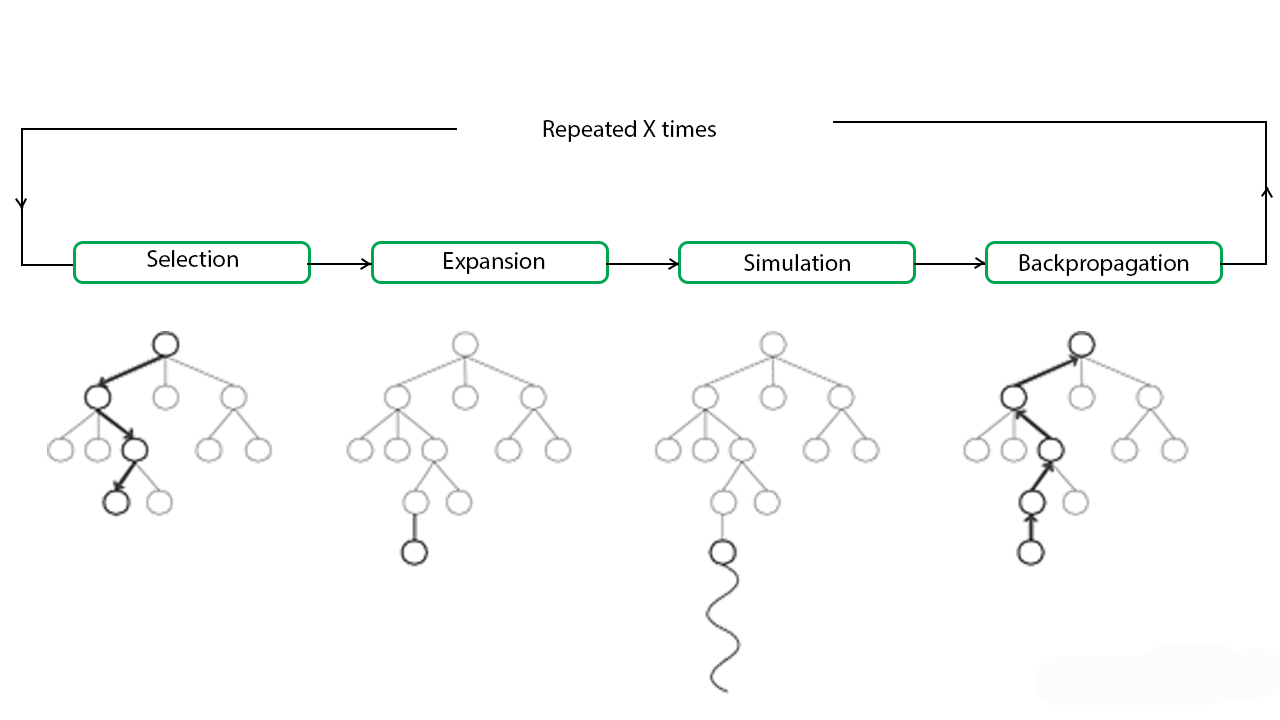Related Articles
ML | Monte Carlo Tree Search (MCTS)
• Last Updated : 14 Jan, 2019

Monte Carlo Tree Search (MCTS) is a search technique in the field of Artificial Intelligence (AI). It is a probabilistic and heuristic driven search algorithm that combines the classic tree search implementations alongside machine learning principles of reinforcement learning.

In tree search, there’s always the possibility that the current best action is actually not the most optimal action. In such cases, MCTS algorithm becomes useful as it continues to evaluate other alternatives periodically during the learning phase by executing them, instead of the current perceived optimal strategy. This is known as the ” exploration-exploitation trade-off “. It exploits the actions and strategies that is found to be the best till now but also must continue to explore the local space of alternative decisions and find out if they could replace the current best.

Exploration helps in exploring and discovering the unexplored parts of the tree, which could result in finding a more optimal path. In other words, we can say that exploration expands the tree’s breadth more than its depth. Exploration can be useful to ensure that MCTS is not overlooking any potentially better paths. But it quickly becomes inefficient in situations with large number of steps or repetitions. In order to avoid that, it is balanced out by exploitation. Exploitation sticks to a single path that has the greatest estimated value. This is a greedy approach and this will extend the tree’s depth more than its breadth. In simple words, UCB formula applied to trees helps to balance the exploration-exploitation trade-off by periodically exploring relatively unexplored nodes of the tree and discovering potentially more optimal paths than the one it is currently exploiting.
For this characteristic, MCTS becomes particularly useful in making optimal decisions in Artificial Intelligence (AI) problems.

Monte Carlo Tree Search (MCTS) algorithm:
In MCTS, nodes are the building blocks of the search tree. These nodes are formed based on the outcome of a number of simulations. The process of Monte Carlo Tree Search can be broken down into four distinct steps, viz., selection, expansion, simulation and backpropagation. Each of these steps is explained in details below:

• Selection: In this process, the MCTS algorithm traverses the current tree from the root node using a specific strategy. The strategy uses an evaluation function to optimally select nodes with the highest estimated value. MCTS uses the Upper Confidence Bound (UCB) formula applied to trees as the strategy in the selection process to traverse the tree. It balances the exploration-exploitation trade-off. During tree traversal, a node is selected based on some parameters that return the maximum value. The parameters are characterized by the formula that is typically used for this purpose is given below.where;
Si = value of a node i
xi = empirical mean of a node i
C = a constant
t = total number of simulations

When traversing a tree during the selection process, the child node that returns the greatest value from the above equation will be one that will get selected. During traversal, once a child node is found which is also a leaf node, the MCTS jumps into the expansion step.
• Expansion: In this process, a new child node is added to the tree to that node which was optimally reached during the selection process.
• Simulation: In this process, a simulation is performed by choosing moves or strategies until a result or predefined state is achieved.
• Backpropagation: After determining the value of the newly added node, the remaining tree must be updated. So, the backpropagation process is performed, where it backpropagates from the new node to the root node. During the process, the number of simulation stored in each node is incremented. Also, if the new node’s simulation results in a win, then the number of wins is also incremented.

The above steps can be visually understood by the diagram given below:These types of algorithms are particularly useful in turn based games where there is no element of chance in the game mechanics, such as Tic Tac Toe, Connect 4, Checkers, Chess, Go, etc. This has recently been used by Artificial Intelligence Programs like AlphaGo, to play against the world’s top Go players. But, its application is not limited to games only. It can be used in any situation which is described by state-action pairs and simulations used to forecast outcomes.

Pseudo-code for Monte Carlo Tree search:

 `# main function for the Monte Carlo Tree Search ` `def` `monte_carlo_tree_search(root): ` `     `  `    ``while` `resources_left(time, computational power): ` `        ``leaf ``=` `traverse(root)  ` `        ``simulation_result ``=` `rollout(leaf) ` `        ``backpropagate(leaf, simulation_result) ` `         `  `    ``return` `best_child(root) ` ` `  `# function for node traversal ` `def` `traverse(node): ` `    ``while` `fully_expanded(node): ` `        ``node ``=` `best_uct(node) ` `         `  `    ``# in case no children are present / node is terminal  ` `    ``return` `pick_univisted(node.children) ``or` `node  ` ` `  `# function for the result of the simulation ` `def` `rollout(node): ` `    ``while` `non_terminal(node): ` `        ``node ``=` `rollout_policy(node) ` `    ``return` `result(node)  ` ` `  `# function for randomly selecting a child node ` `def` `rollout_policy(node): ` `    ``return` `pick_random(node.children) ` ` `  `# function for backpropagation ` `def` `backpropagate(node, result): ` `    ``if` `is_root(node) ``return` `    ``node.stats ``=` `update_stats(node, result)  ` `    ``backpropagate(node.parent) ` ` `  `# function for selecting the best child ` `# node with highest number of visits ` `def` `best_child(node): ` `    ``pick child with highest number of visits `

As we can see, the MCTS algorithm reduces to a very few set of functions which we can use any choice of games or in any optimizing strategy.

Advantages of Monte Carlo Tree Search:

1. MCTS is a simple algorithm to implement.
2. Monte Carlo Tree Search is a heuristic algorithm. MCTS can operate effectively without any knowledge in the particular domain, apart from the rules and end conditions, and can can find its own moves and learn from them by playing random playouts.
3. The MCTS can be saved in any intermediate state and that state can be used in future use cases whenever required.
4. MCTS supports asymmetric expansion of the search tree based on the circumstances in which it is operating.

Disadvantages of Monte Carlo Tree Search:

1. As the tree growth becomes rapid after a few iterations, it requires a huge amount of memory.
2. There is a bit of a reliability issue with Monte Carlo Tree Search. In certain scenarios, there might be a single branch or path, that might lead to loss against the opposition when implemented for those turn-based games. This is mainly due to the vast amount of combinations and each of the nodes might not be visited enough number of times to understand its result or outcome in the long run.
3. MCTS algorithm needs a huge number of iterations to be able to effectively decide the most efficient path. So, there is a bit of a speed issue there.My Personal Notes arrow_drop_up
Recommended Articles
Page :